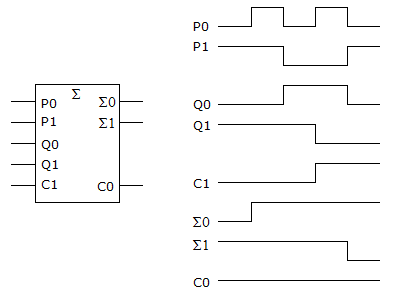# Digital Electronics - Digital Arithmetic Operations and Circuits

### Exercise :: Digital Arithmetic Operations and Circuits - General Questions

46.

Convert the decimal numbers 275 and 965 to binary-coded decimal (BCD) and add. Select the BCD code groups that reflect the final answer.

 A. 1101 1110 1010 B. 1110 1010 1110 C. 0001 0010 0100 0000 D. 0010 0011 0100 0000

Answer: Option C

Explanation:

No answer description available for this question. Let us discuss.

47.

When multiplying 13 × 11 in binary, what is the third partial product?

 A. 1011 B. 00000000 C. 100000 D. 100001

Answer: Option B

Explanation:

No answer description available for this question. Let us discuss.

48.

How many BCD adders would be required to add the numbers 97310 + 3910?

 A. 3 B. 4 C. 5 D. 6

Answer: Option A

Explanation:

No answer description available for this question. Let us discuss.

49.

The selector inputs to an arithmetic/logic unit (ALU) determine the:

 A. selection of the IC B. arithmetic or logic function C. data word selection D. clock frequency to be used

Answer: Option B

Explanation:

No answer description available for this question. Let us discuss.

50.

What is wrong, if anything, with the circuit in the given figure based on the logic analyzer display accompanying the circuit?A. The CO terminal is shorted to ground. B. The S1 output is shorted to Vcc. C. The P1 input is not being added into the total. D. Nothing is wrong; the circuit is functioning correctly.

Answer: Option C

Explanation:

No answer description available for this question. Let us discuss.

#### Current Affairs 2021

Interview Questions and Answers